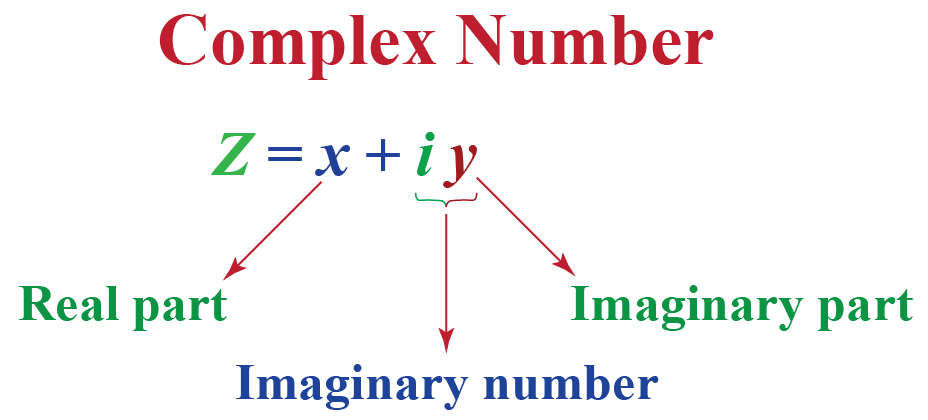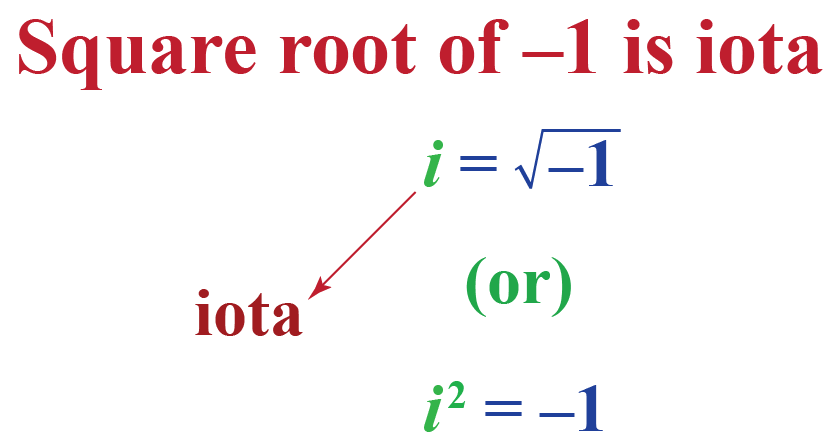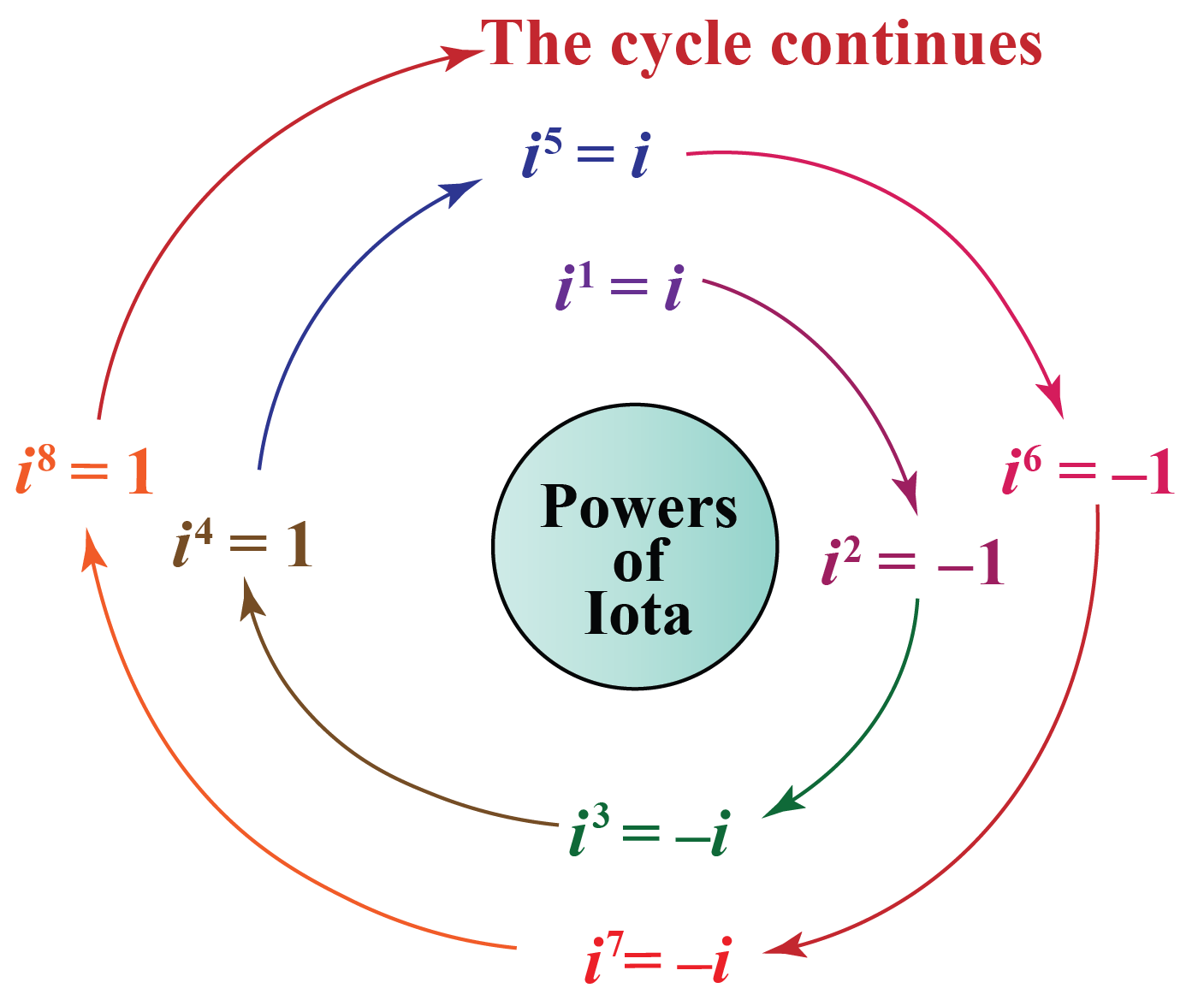# Imaginary Numbers

Imaginary Numbers

complex number in standard form is $$x+iy$$ and is usually represented by $$z$$.

Here both $$x$$ and $$y$$ are real numbers.

• $$x$$ is called the real part which is denoted by $$Re(z)$$.
• $$y$$ is called the imaginary part $$Im(z)$$.
• $$iy$$ is an imaginary number.In other words, a complex number is one that includes both real and imaginary parts.

The notation $$i$$ is the foundation for all imaginary numbers.

Imaginary numbers, as the name says, are numbers not real.

Let's explore more about imaginary numbers.

In this lesson, you will learn about complex numbers, imaginary numbers examples,  imaginary numbers operations, imaginary numbers rules, roots of complex numbers, pure imaginary number, complex number in standard form, simplifying imaginary numbers

## Lesson Plan

 1 What Is the Definition Of Imaginary Numbers? 2 Tips and Tricks 3 Important Notes on Imaginary Numbers 4 Solved Examples on Imaginary Numbers 5 Interactive Questions on Imaginary Numbers

## What Is the Definition Of Imaginary Numbers?

An imaginary number is a number that results in a negative number when it is squared.

Imaginary numbers are defined as the square root of the negative numbers where it does not have a definite value.

Let's try squaring some real numbers:

\begin{align} (-2)^2 &= -2 \times -2 =4\\[0.2cm] 5^2 &= 5 \times 5 =25\\[0.2cm] (1.3)^2&= 1.3 \times 1.3 =1.69 \end{align}

Is any of the result a negative number?

No.

It means the square of any real number is always positive.

Then what number results in a negative square? It is an imaginary number.

An imaginary number is the product of a real number and iota $$(i)$$, the imaginary unit.

Here are some imaginary numbers examples:

\begin{align} 3i &= 3 \sqrt{-1}= \sqrt{9} \times \sqrt{-1}= \sqrt{-9}\\[0.2cm] \sqrt{3}i &= \sqrt{3} \times \sqrt{-1}= \sqrt{-3} \end{align}

So in general:

 $$\sqrt{-x} = i \sqrt{x}$$Tips and Tricks
1. When the minus sign comes out of the square root, it becomes $$i$$.
2. $$i$$ is used to simplify $$\sqrt[n]{x}$$ where $$n$$ is an even number and $$x$$ is a negative number.
3. To calculate the negative powers of iota, we use the rule $$\mathbf{\dfrac{1}{i}=-i}$$
4. To find any power of iota, say $$\mathbf{i^n}$$, just divide $$\mathbf{n}$$ by 4 and find the remainder, r.

Then just apply $$\mathbf{i^n=i^r}$$.

Here, you just need to remember two things $$\mathbf{i^2=-1}$$ and $$\mathbf{i^3=-i}\ ## What Are the Rules Of Imaginary Numbers?Let us see how to calculate some other powers of iota. $\begin{array}{l} i^{3}=i \times i^{2}=i \times-1=-i \\ i^{4}=i^{2} \times i^{2}=-1 \times-1=1 \\ \end{array}$So, the generalized imaginary numbers rules are: $\begin{array}{l}i^{4 k}=1 \\i^{4 k+1}=i \\i^{4 k+2}=-1 \\i^{4 k+3}=-i\end{array}$ Here, \(k$$ is any whole number.

One needs to be aware of certain rules for simplifying imaginary numbers.

Let's have a look at how to find the value of imaginary numbers using these rules!

Let us take an example: $$6i$$

Where

$$6$$ is the real number and i is the imaginary unit.

Putting the vaue of $$i$$ in $$6i$$, we will get $$6 \sqrt{-1}$$.

Another example: $$(4 i)^{2}$$

$$4$$ is the real number and i is the imaginary unit.

When this number $$4i$$ is squared, we will get the negative result as -$$16$$.

## What Are the Operations Of Imaginary Numbers?

In Mathematics, the basic arithmetic operations are addition, subtraction, multiplication, and division.

Also, we know that imaginary numbers are a part of complex numbers.

Let's discuss imaginary number operations.

• Addition or subtraction: The sum or difference of two complex numbers is a complex number whose real part and the imaginary part are obtained by just adding or subtracting the corresponding real parts and imaginary parts respectively. i.e.,
$$(a+ib)\pm(x+iy)$$= $$(a\pm x)+i(b\pm y)$$
• Multiplication: The product of two complex numbers is found by multiplying them considering them as binomials. i.e.,
\begin{align} (a+ib)(x+iy)= ax+iay+ibx+i^2by\\[0.2cm] =ax+i(ay+bx)-by\,[\because i^2=-1]\\[0.2cm] = (ax-by)+ i (ay+bx) \end{align}
• Division: To divide two complex numbers, we rationalize the denominator by multiplying and dividing by the conjugate of the complex number. \begin{align} &\dfrac{a+ib}{x+iy}\\[0.2cm] &=\dfrac{a+ib}{x+iy} \cdot \dfrac{x-iy}{x-iy}\\[0.2cm] &= \dfrac{ax-aiy+ibx+by}{x^2+y^2}\,\,\,\, [\because i^2=-1]\\[0.2cm] &= \dfrac{(ax+by)+i(bx-ay)}{x^2+y^2} \end{align}

Here are a few activities for you to practice.

## Let's Summarize

The mini-lesson targeted the fascinating concept of Imaginary Numbers. The math journey around imaginary numbers starts with what a student already knows, and goes on to creatively crafting a fresh concept in the young minds. Done in a way that not only is relatable and easy to grasp, but also will stay with them forever. Here lies the magic with Cuemath!

At Cuemath, our team of math experts is dedicated to making learning fun for our favorite readers, the students!

Through an interactive and engaging learning-teaching-learning approach, the teachers explore all angles of a topic.

Be it worksheets, online classes, doubt sessions, or any other form of relation, it’s the logical thinking and smart learning approach that we at Cuemath believe in.

### 1. What are imaginary numbers?

An imaginary number is a complex number written as a real number multiplied by the imaginary unit i.

### 2. Is 0 an imaginary number?

Since an imaginary number is the square root of a nonpositive real number. And zero is nonpositive and is its own square root, so zero can be considered as an imaginary number.

### 3. What are real and imaginary numbers?

The set of Real Numbers is the union of the set of Rational Numbers Q and the set of Irrational Numbers I. The set of real numbers is denoted by R.

Imaginary numbers are complex numbers only, written as a real number multiplied by the imaginary unit i.

### 4. What is an imaginary number equal to?

Imaginary numbers are equal to real numbers multiplied by 'i'.

### 5. Are imaginary numbers positive or negative?

Imaginary Numbers are neither positive nor negative.

### 6. What do you mean by pure imaginary numbers?

Any complex number which has its real part equal to 0, is called a pure imaginary number.

### 7. What type of number is real imaginary or complex?

A complex number, in its standard form, can be expressed as a + bi, a being the real part & bi being the imaginary part.

### 8. What is the number i equal to?

The imaginary unit 'i' is equal to the square root of -1

### 9. How do you add imaginary numbers?

In order to add, just add the corresponding real and imaginary parts of the given complex numbers.

### 10. Is every number a complex number?

Every real number is a complex number, whereas every complex number is not necessarily a real number.

More Important Topics
Numbers
Algebra
Geometry
Measurement
Money
Data
Trigonometry
Calculus
More Important Topics
Numbers
Algebra
Geometry
Measurement
Money
Data
Trigonometry
Calculus
Learn from the best math teachers and top your exams

• Live one on one classroom and doubt clearing
• Practice worksheets in and after class for conceptual clarity
• Personalized curriculum to keep up with school